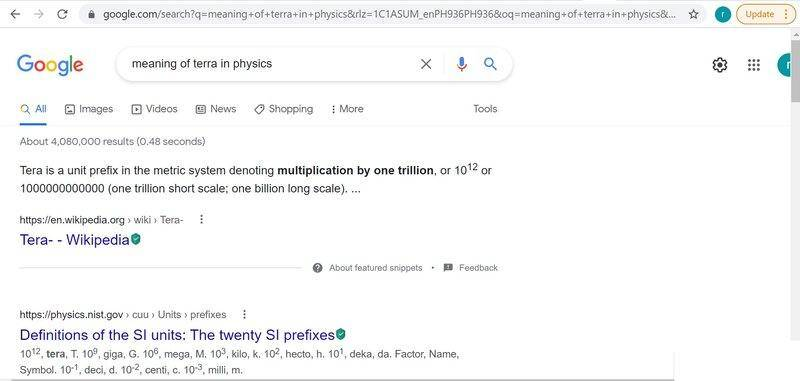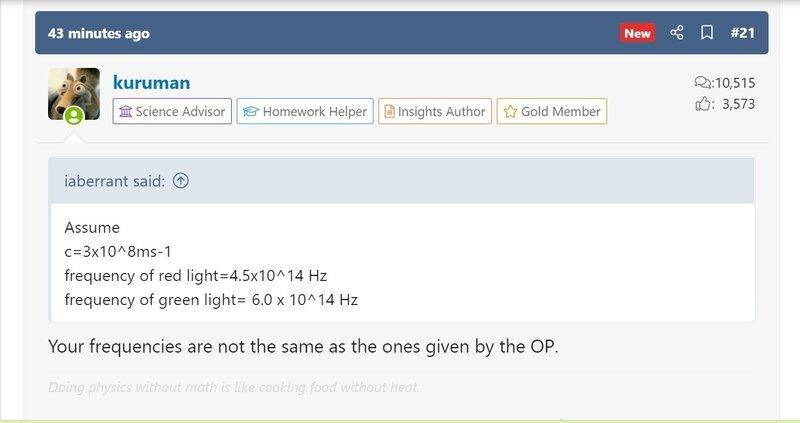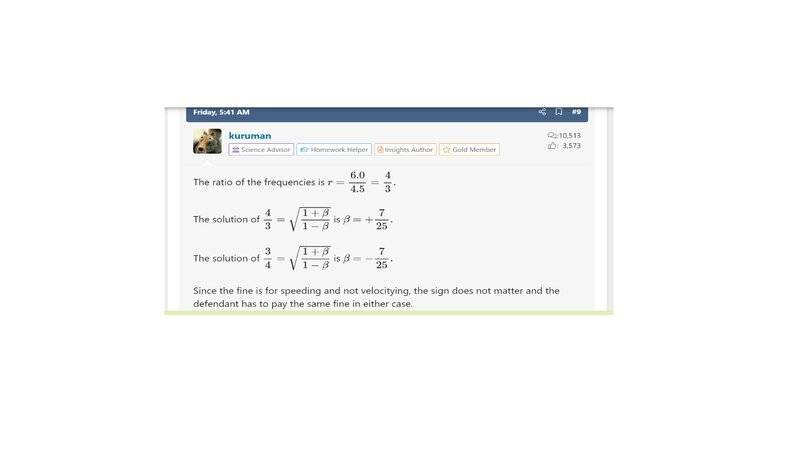# Special Relativity Problem -- Speeding through a red light to make it look green

By now i must be driving you guys crazy with my relativity questions , but this is my last one! i swear! =]

## Homework Statement

A noted physicist was stopped by police for travelling through a red traffic light. The physicist argued that to because he was travelling fast, the colour of the light had changed from the red wavelength to that of a green wavelength

The judge said that he would let him off the charge of running the red light, but would fine him for speeding at the rate of one cent for every 100km/hr he was travelling over 100km/hr

Was he fined? and how much was his fine?

Assume
c=3x10^8ms-1
frequency of red light=4.5x10^14 Hz
frequency of green light= 6.0 x 10^14 Hz

## Homework Equations

Would this problem also be related to the Dopper Shifty as the change of colour of the traffic lights? And therefore would the doppler shift be appropriate to use in this case ? But i have come across a relativistic doppler formula; f/f0 = √[(1 + v/c)/(1 - v/c)] ?

there is a non relativistic doppler formula ?
hence ;
∆f = f0*(v/c)

v = ∆f/f0 * c

where ;
∆f = 1.5*10^14
f0 = 4.5*10^14
v = 0.33 c

How can i go about this problem and any other ideas of what is involved in solving the problem ?

According to our modern day understanding of physics, light is neither a wave nor a particle. Sometimes, light behaves as a wave, sometimes as a particle, but not both at the same time. Light, as a wave that exhibits wave motion, is a periodic motion characterized, among other things, by a wavelength ##λ~##, a period ##T_0~##, and a wave frequency ##ν~##.
##\dots~λ~\Rightarrow~\begin{cases}\begin{align} & \text {the distance traveled by the wave along the } \nonumber \\
& \text {direction of propagation in one period or one cycle} \nonumber \\
& \text {of the motion before the next cycle is repeated}~\dots\nonumber \end{align}\end{cases}##
##\dots~T_0~\Rightarrow~\begin{cases}\begin{align} & \text {the time to complete one cycle and travel } \nonumber \\ & \text { the distance of one wavelength }~ λ~\dots\nonumber \end{align}\end{cases}##
##\dots~ν~\Rightarrow~\begin{cases}\begin{align} & \text { tells the number of waves passing } \nonumber \\ & \text {through a given point in a given time }~\dots\nonumber \end{align}\end{cases}##
The larger the period ##T_0## (the longer it takes for one complete cycle to pass through), the longer the wavelength ##λ~##, and the smaller the frequency ##ν## (the fewer the number of full cycles that pass through). Being more familiar with length in the Special Theory of Relativity, we consider the wavelength of light in this problem instead of the frequency.
##\dots~## for red light ##~\Rightarrow~##average ##~λ_\rm R## = 685##×10^{-9}## m = 685 nm##~\dots~##
##\dots~## for green light ##~\Rightarrow~##average ##~λ_\rm G## = 533##×10^{-9}## m = 533 nm##~\dots~##
Red light becoming green with ##~λ_\rm R~\gt~λ_\rm G~\Rightarrow~##length contraction##~\dots~##
##\Rightarrow~λ_\rm G## = ##λ_\rm R[1 - (v/c)^2]^{1/2}\Rightarrow~1 - (v/c)^2~##= ##(λ_\rm G/λ_\rm R)^2~##= 0.605443##~\dots~##
##\Rightarrow~(v/c)~##=##~(0.394557)^{1/2}~##= 0.628##~\Rightarrow~v~##= ##(0.628)c~\Rightarrow~v~##=##~1.884 ×10^8~\rm m ⋅ \rm s^{-1}~##...
##\Rightarrow~v~##=##~6.7824 ×10^8~\rm km ⋅ \rm hr^{-1}~\Rightarrow~v/(100~\rm km ⋅ \rm hr^{-1})~##=##~6.7824 ×10^6~##gives the number of times in excess of the 100##~\rm km ⋅ \rm hr^{-1}~##speed limit if the red traffic light appears green to the driver.
Being charged of a fine at the rate of one cent for every 100##~\rm km ⋅ \rm hr^{-1}~##he was traveling over 100##~\rm km ⋅ \rm hr^{-1}~##, the driver must pay a total fine of almost $68,000. berkeman Mentor the driver must pay a total fine of almost$68,000.
@kumusta -- Because this thread is over 10 years old, it is okay for you to post a solution. Just keep in mind that for current schoolwork/homework threads, solutions cannot be provided by us. The student must do the bulk of the work. Thanks.

Everything clear. No solutions for current schoolwork/homework threads.

•berkeman
Thanks too.

kuruman
Homework Helper
Gold Member
For the record, the number provided by @kumusta does not use the relativistic Doppler shift equation. The answer I got with ##\beta = v/c=\frac{7}{25}## from using the equation ##\dfrac{f_{\text{source}}}{f_{\text{receiver}}}=\sqrt{\dfrac{1+\beta}{1-\beta}}## is $30,200 plus change. ••hutchphd, PeroK and berkeman My solution took into account the relativistic effect of length contraction only, if it could be called as such. I failed to consider the fact that time is not absolute but is generally different for different observers. So obviously, the solution that I gave cannot be the correct one. Many thanks to kuruman for pointing it out. •berkeman and kuruman While looking at kuruman's post #6, I noticed that since ##(1 - β) < (1 + β)~##, then he must have used the frequency of green light for ##f_\rm {source}~##and the frequency of red light for ##f_\rm {receiver}~##: ... the answer I got with ##\beta = v/c=\frac{7}{25}## from using the equation ##\dfrac{f_{\text{source}}}{f_{\text{receiver}}}=\sqrt{\dfrac{1+\beta}{1-\beta}}## is$30,200 plus change.
I think it should be the other way around since the source, which is the traffic light, must have shown red and not green. I suspect now that there's also something wrong with kuruman's computations.
Anyway, the answer ##~v = (0.628)c~## that I got must be rather high. Knowing the fact that frequency and wavelength are related by the equation ##~v = fλ = λ/T~##, taking relativistic time dilation into account too, with larger value of ##~T~##, aside from length contraction, would clearly give a smaller value for ##~v~## than the one which treats time as an absolute quantity.

•PeroK
kuruman
Homework Helper
Gold Member
The ratio of the frequencies is ##r=\dfrac{6.0}{4.5}=\dfrac{4}{3}.##

The solution of ##\dfrac{4}{3}=\sqrt{\dfrac{1+\beta}{1-\beta}}## is ##\beta=+\dfrac{7}{25}.##

The solution of ##\dfrac{3}{4}=\sqrt{\dfrac{1+\beta}{1-\beta}}## is ##\beta=-\dfrac{7}{25}.##

Since the fine is for speeding and not velocitying, the sign does not matter and the defendant has to pay the same fine in either case.

berkeman
Mentor
velocitying
TIL a new word at PF...•••SammyS, DaveC426913, jbriggs444 and 1 other person
kuruman
Homework Helper
Gold Member
TIL a new word at PF...velocity [ vuh-los-i-tee ]
noun, plural ve·loc·i·ties
rapidity of motion or operation; swiftness;
a high wind velocity

verb (used without object), ve·loc·i·tyed, ve·loc·i·ty·ing
to move in a fixed direction
we velocitied from the Northside to the Southside in record time

•berkeman
berkeman
Mentor
You using the Urban Dictionary again? This is what I get with Google...kuruman
Homework Helper
Gold Member
You using the Urban Dictionary again? This is what I get with Google...View attachment 289177
Truth be told not even the Urban Dictionary has the word as a verb, I looked. I made it up initially for post #9 and then came up with a dictionary-style definition after seeing your post #10. Hey, that's how the language evolves, no? If enough people use a word in a new context, it will find its way into the real dictionaries.

•berkeman
For the record, it appears that velocitying is the name of a YouTube channel.
View attachment 289187
It's been in YouTube since February 2007?But post #9 was made only yesterday, Thu16Sept2021
Truth be told not even the Urban Dictionary has the word as a verb, I looked. I made it up initially for post #9 and then came up with a dictionary-style definition after seeing post #10 ...

#### Attachments

jbriggs444
Homework Helper
You cannot copyright this word. At least in the U.S. The doctrine of merger applies. In the U.S. you are allowed to copyright the expression of an idea (i.e. the book "Huckleberry Finn") but not the idea of a child floating down a river on a raft with a slave. If there are only a limited number of ways to express an idea, the expression merges with the idea and the expression becomes uncopyrightable.

Trademark, however, follows a different standard. Which explains how the phrase "Super Bowl" can be trademarked, even though it would not be copyrightable.

•hutchphd
It's okay if that is so. But I think no one has the right to claim just a couple of days ago that a new word originated from him when that particular word already existed more than 10 years ago.

kuruman
Homework Helper
Gold Member
It's okay if that is so. But I think no one has the right to claim just a couple of days ago that a new word originated from him when that particular word already existed more than 10 years ago.
I assume you are referring to my posts #11 and #13.

Firstly, I never claimed that I originated a new word. The word "velocity" has existed as a noun for centuries (velocitas in Latin). I acknowledged so explicitly in #11 by showing the existing noun and, beneath it, the verb "to velocity" and then again in #13 where I stated "If enough people use a word in a new context, ##\dots~##"

Secondly, what I made up from the word "velocity" is its use as a verb. To that end, I provided a dictionary-like definition complete with past tense to show that the verb is regular. I included a sentence to illustrate its use in a sentence as an intransitive verb without an object.

Thirdly, the use of "velocitying" in YouTube is irrelevant and only superficially akin to the verb defined in #11, but it is not a verb or part thereof. It is the name of a playlist, therefore a noun, and conveys no action. An example of its use in a sentence might be, "Velocitying is a YouTube playlist" in which, as a noun, it serves as the subject of the copulative verb "is".

Fourthly, I believe that the original question posed by the OP has been fully, albeit belatedly, answered therefore I will henceforth cease and desist posting more silliness on this revenant thread.

••hutchphd and jbriggs444
I'm sorry but I disagree with this assesment in post #17 regarding this thread:
... I believe that the original question posed by the OP has been fully .. answered ...
The solutions given in post #6 and #9 are somewhat arbitrary and has some inconsistencies:
The ratio of the frequencies is ##r=\dfrac{6.0}{4.5}=\dfrac{4}{3}.##
The solution of ##\dfrac{4}{3}=\sqrt{\dfrac{1+\beta}{1-\beta}}## is ##\beta=+\dfrac{7}{25}.##
The solution of ##\dfrac{3}{4}=\sqrt{\dfrac{1+\beta}{1-\beta}}## is ##\beta=-\dfrac{7}{25}.##
Since the fine is for speeding and not velocitying, the sign does not matter and the defendant has to pay the same fine in either case.
I pointed these things out in post #8:
While looking at kuruman's post #6, I noticed that since ##(1 - β) < (1 + β)~##, then he must have used the frequency of green light for ##f_\rm {source}~##and the frequency of red light for ##f_\rm {receiver}~##:
I think it should be the other way around since the source, which is the traffic light, must have shown red and not green. I suspect now that there's also something wrong with kuruman's computations.
It seems that no attention was paid to my misgivings and they only fell on deaf ears.
Without knowing the correct solution then, I would have just forgotten it and left things as they were. But just recently, I think I found the correct solution and I would like to share it to others.
I'll send the right solution in the next post.

kuruman
Homework Helper
Gold Member
t seems that no attention was paid to my misgivings and they only fell on deaf ears.
Without knowing the correct solution then, I would have just forgotten it and left things as they were. But just recently, I think I found the correct solution and I would like to share it to others.
I'll send the right solution in the next post.
Our ears are not deaf. I can only speak for myself when I say that if I have nothing to add to what I have already said, I say nothing. I will make an exception and repeat myself except in more detail. Start with $$\frac{f_{\text{source}}}{f_{\text{receiver}}}=\sqrt{\frac{1+\beta}{1-\beta}}.$$Obviously it's the ratio of frequencies that matters. Looking at the numbers, the lower frequency is red. Thus, if ##f_{\text{source}}=3##, then ##f_{\text{receiver}}=4.## The source is approaching the receiver, which means that ##\beta## is negative. To ensure that, we replace ##\beta## with ##-|\beta|## in the equation and then solve to find the relative speed:$$\frac{f_{\text{source}}}{f_{\text{receiver}}}=\sqrt{\frac{1-|\beta|}{1+|\beta|}}=\frac{3}{4}.$$ If you solve that equation, you get ##|\beta|=\frac{7}{25}.## The point I tried to make earlier is that whether you write the frequency ratio as 3/4 or 4/3, you will get ##|\beta|=7/25## and the fine is the same.

The correct formula for relativistic Doppler shift can be found in Fowles's book ##\text {Intro. to Modern Optics}~##, 2nd Ed.,©1975. It says there that the observed frequency (frequency received) ##~f'~## is given by f' = f \sqrt { \frac {1 - β } {1 + β } }~\Leftarrow~\begin{cases} {\begin{align} ~& {\rm {either} }~β\gt0~\text {if the source and receiver } \nonumber \\ & \text {are moving away from one another} ~\dots \nonumber \\ & {\rm {or} }~β\lt0~\text {if they are moving toward each other}~\dots \nonumber\end{align} }\end{cases} where ##~β = v/c~.~##The special theory of relativity removed the distinction between observer in motion and source in motion so that what is important now is only the relative motion between them. Notice that when the source and the receiver are moving away from each other so that ##~β\gt0~##, then ##~1 + β \gt 1 - β~## which gives ##f'~\lt~f~## that signifies a decrease in the observed frequency and a corresponding increase in the wavelength ##\lambda~##. Frequency and wavelength are inversely proportional
to each other, meaning that if one of them increases, then the other decreases.
In the particular instance of an overspeeding driver traveling through a red traffic light, we have the second case in the relativistic formula mentioned above, namely the one where the source and receiver are moving toward one another. The correct formula to apply is the one with ##~β\lt0~## so we make the replacement ##~β = -v/c~## and get f' = f \sqrt { \frac {1 + v/c } {1 - v/c } }~\Leftarrow~\begin{cases} {\begin{align} ~& [1 + v/c~] \gt [1 - v/c~]~\text {indicating that} \nonumber \\ & f'\gt f,\text {showing an increase in frequency} \nonumber \\ & \text {that shortens the wavelength}~\dots\nonumber\end{align} }\end{cases}Solving for ##~v~## we obtain ##~v = c[~(f'/f)^2 - 1~]/[~(f'/f)^2 + 1~]~\dots##
Substituting the values for the average frequency of green and red colors of light: $$\dots~f_{\rm green} = 565~{\rm THz} = f'~\dots~f_{\rm red} = 440~{\rm THz} = f~\dots$$ $$\dots~v = (0.245)c = 7.35~×~10^7~{\rm m} · {\rm s}^{-1} = 2.646~×~10^8~{\rm km} · {\rm hr}^{-1}~\dots$$ \dots~ \begin{cases}\begin{align} & \text { the number of times in excess }\nonumber \\ & \nonumber \text { of the 100 km} · {\rm {hr}^{-1}}~{\rm {speed} }~\Rightarrow~2.646~×~10^6\nonumber \end{align}\end{cases} Almost \$26,500 fine must be paid for one cent every time the 100 km/hr speed limit is exceeded.

kuruman
Homework Helper
Gold Member
Assume
c=3x10^8ms-1
frequency of red light=4.5x10^14 Hz
frequency of green light= 6.0 x 10^14 Hz
Your frequencies are not the same as the ones given by the OP.

@kuruman ... Of course they are, they both have the same order of magnitudeThere are minimum and maximum values of the frequency for any given color. I used the average value.

You said in post #21:
Your frequencies are not the same as the ones given by the OP.I posted in #20 to which post #21 was referring to:
$$\dots~f_{\rm green} = 565~{\rm THz} = f'~\dots~f_{\rm red} = 440~{\rm THz} = f~\dots$$
I replied in post #22 to answer #21:
@kuruman ... Of course they are, they both have the same order of magnitude
View attachment 289458
There are minimum and maximum values of the frequency for any given color. I used the average value.
##\text {terra} = 10^{12}##

jbriggs444
Homework Helper
Of course they are, they both have the same order of magnitude
Are you claiming that two numbers are equal if they share the same order of magnitude? Most traffic court judges would disagree.

@kuruman again ... Your post #9 said:
The ratio of the frequencies is ##r=\dfrac{6.0}{4.5}=\dfrac{4}{3}.##
The solution of ##\dfrac{4}{3}=\sqrt{\dfrac{1+\beta}{1-\beta}}## is ##\beta=+\dfrac{7}{25}.##
The solution of ##\dfrac{3}{4}=\sqrt{\dfrac{1+\beta}{1-\beta}}## is ##\beta=-\dfrac{7}{25}.##
Since the fine is for speeding and not velocitying, the sign does not matter and the defendant has to pay the same fine in either case.There you mentioned nothing about ##|β|~,## that ##β## can be ##\pm~.## Then, suddenly in post #19, only after 10 messages had already been posted, you surprisingly come up with the idea that ##β~,## in the formula you used in #9 , can be ##\pm~.## Why didn't you post #19 much earlier or come up with the idea of ##\pmβ~## somewhat sooner so that you could have informed us forum viewers about it for even just a couple of days ago already, and not just now belatedly after I started writing my post #20. It takes me quite a long time to finish writing my posts, and I know that forum mentors have the special privilege of viewing what ordinary forum members are writing in a thread. If ordinary forum viewers use PhysicsForums' platforms to chat only with their friends about things that have nothing to do with physics, then the PhysicsForums' administrative staff and mentors have all the right to do something to avoid their platforms being misused and abused. But some sort of privacy must also be allowed to forum viewers only while they are writing their posts to answering a thread. But exactly how, I cannot make any suggestion. Also, I think some sort of checks and counter checks must be used to prevent those with special privileges to abuse the special treatment given to them.